HOME / Applications / Transformer Simulation - Perform open and short circuit tests easily inside SolidWorks

# Transformer Simulation - Perform open and short circuit tests easily inside SolidWorks

Used Tools:## Transformer

A transformer is a static electrical machine that transfers electrical energy between 2 or more circuits through the principle of electromagnetic induction. As shown in Figure 1, the transformer consists of a core (usually laminated steel), a primary winding and a secondary winding. A time varying current in the primary coil produces a time varying magnetic field. This time varying magnetic field induces a voltage in the secondary coil. This is due to the principle of Faraday’s law of induction. So, power can be easily transferred from one circuit to the other without physical contact. So why is this important.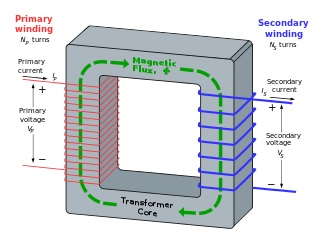Figure 1 - A single phase transformer

## Applications of transformers

Transformers find useful application in electric power industry. In electric power applications, transformers are used to increase or decrease the AC voltage. Since the adoption of AC power, transformers have become ubiquitous in electrical power transmission and distribution industry. Transformers are also used in electronic and RF industry and hence they vary in size. The smallest transformers used in RF industry is of the order of few cubic centimeters and high power transformers used to interconnect power grids can be in the order of few cubic meters and can weight several tons.

## Losses in transformers

There are 2 main kinds of losses in a transformer that are useful for engineers.

• Core loss
• Winding loss

The objective of a good design is to reduce the losses in the transformer. Once a transformer is designed, engineers build a prototype and then measure the losses using open circuit and short circuit tests. Also, these tests enable engineers to create an equivalent circuit of a transformer. Once you have the equivalent circuit of a transformer it is very easy to replace the transformer by its equivalent circuit and perform system level simulation.

## Open circuit test

The open circuit test, as shown in Figure 2 by its connection diagram, is used to determine the core loss in a transformer. As the name suggests, there is no load in one of the windings (usually the high voltage side of the transformer). The voltage in the low voltage winding is gradually increased till it is equal to the rated voltage of the low voltage circuit. The wattmeter that is connected to the low voltage circuit is used to measure the input power and this value is taken to be the core loss in the transformer.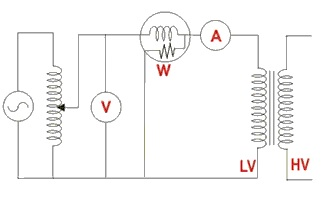Figure 2 - Open circuit test

## Short circuit test

Figure 3 shows the connection diagram of the short circuit test. The low voltage side of the transformer is short circuited. Now in the high voltage side, the voltage is gradually increased till the current reaches the rated current of the high voltage side. The wattmeter reading can be approximated as the copper loss in the transformer. So the short circuit test is used to determine the copper loss in the transformer.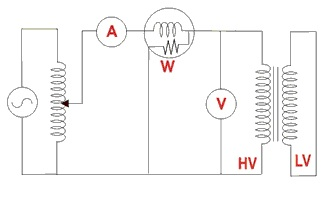Figure 3 - Short circuit test

## Open circuit test and short circuit test simulation

The interesting feature about simulation in EMS is the ability to perform both the above-mentioned tests virtually inside SolidWorks. For the open circuit test, the following inputs are required.

1. Material property of the core – the B-H curve of the steel material, lamination details, Core loss curve for the laminate (P-B curve)
2. Rated voltage in the Low Voltage side must be applied to the low voltage winding
3. The high voltage side must be kept open i.e. a current equal to 0 Amps must be applied to the high voltage winding
Once the simulation is completed, EMS gives the core loss as an output. Once can also get the Low Voltage side current from EMS.
To perform short circuit test simulation the following inputs are required.
1. The low voltage side must be shorted. Hence, we apply a 0 voltage across the low voltage winding.
2. In the high voltage winding we apply different voltages and measure the current till we get the current equal to rated current in the high voltage side. This can be performed using the Parametric simulation in EMS where the applied voltage can be varied and the current can be measured. Then we take the value of the voltage that gives the rated current and perform the short circuit simulation.
Once the simulation is completed, EMS gives the value of the copper loss, leakage inductance and the winding resistance. The results from both the open circuit and short circuit tests is used to create the equivalent circuit of the transformer.

Discussion of results including equivalent circuitIn this section, I will show you briefly the modeling inside EMS and discuss the obtained results. Figure 4 shows the SolidWorks model used for simulation. Figure 5 shows the material used for the laminate. Figure 6 shows the coil definition inside EMS. The results are obtained for both the open and short circuit simulations. In EMS, each test is performed as a separate study. Figure 7 shows the Result table and Figure 8 shows the section plot of the magnetic flux density for the open circuit test.

## Discussion of results including equivalent circuit

In this section, I will show you briefly the modeling inside EMS and discuss the obtained results. Figure 4 shows the SolidWorks model used for simulation. Figure 5 shows the material used for the laminate. Figure 6 shows the coil definition inside EMS. The results are obtained for both the open and short circuit simulations. In EMS, each test is performed as a separate study. Figure 7 shows the Result table and Figure 8 shows the section plot of the magnetic flux density for the open circuit test.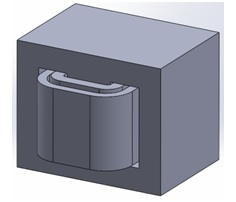Figure 4 - Single phase transformer simulated in EMS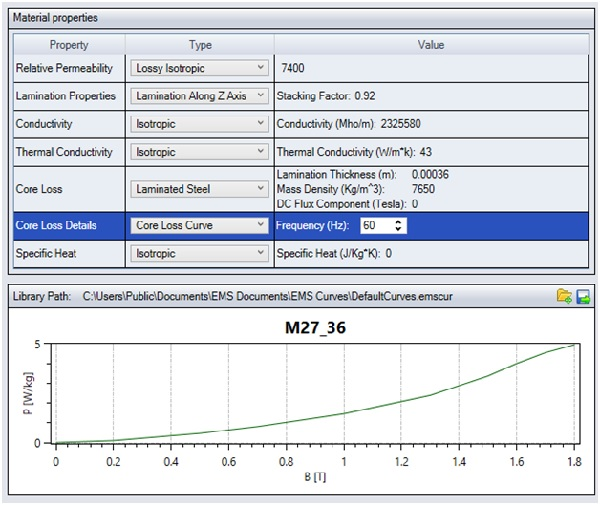Figure 5 - material used for laminates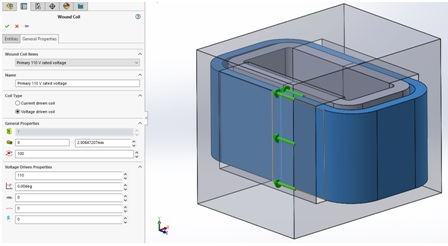Figure 6 - Coil definition inside EMS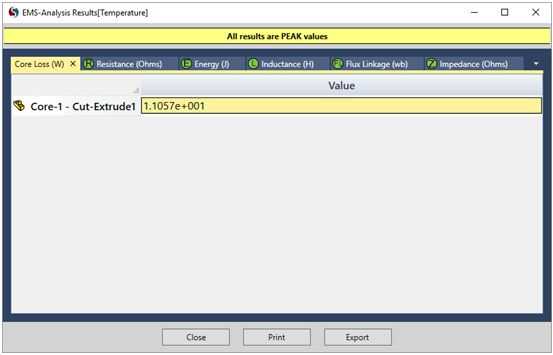Figure 7 - Result table contains all the results including the Core Loss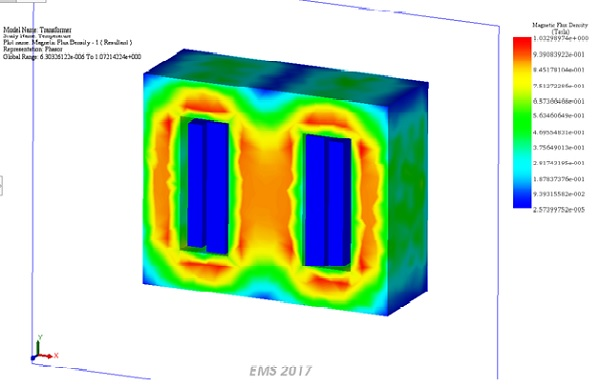Figure 8 - Section plot of the magnetic flux density

## Conclusion

EMS for SolidWorks is a very efficient and handy simulation software where engineers can create 3D geometry of their transformers and simulate both open and short circuit tests. The core loss computed by the open circuit test was 11 Watts and the copper loss computed by the short circuit test were about 188 and 200 W in the primary and secondary coils respectively. Figure 9 shows the final equivalent circuit for the transformer.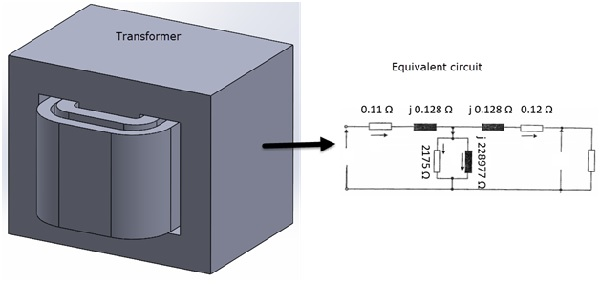Figure 9 - Equivalent circuit of the transformer

## Videos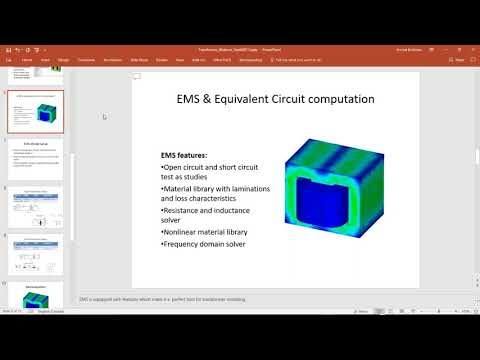#### EMS Webinar: Transformer Losses Computation and Transformer Equivalent Circuit Analysis

Upcoming Webinars